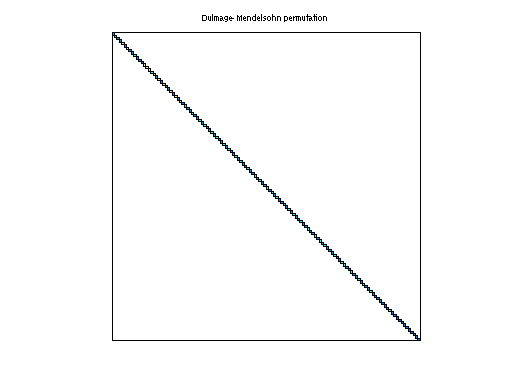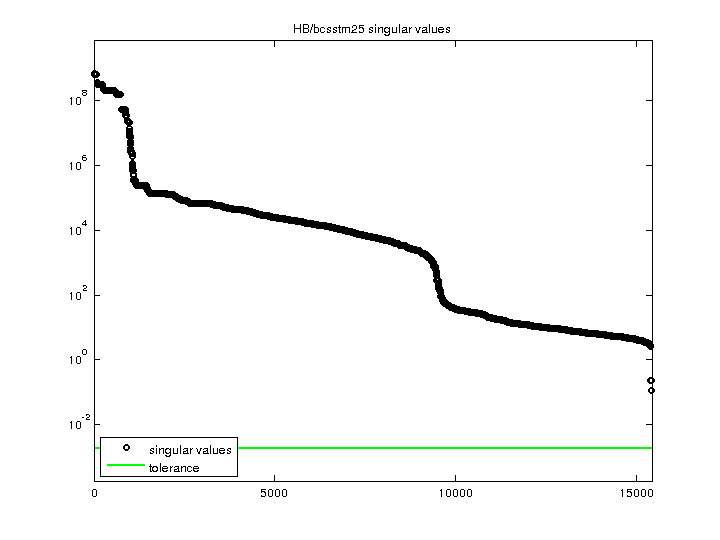Matrix: HB/bcsstm25

Description: SYMMETRIC MASS MATRIX - 76 STORY SKYSCRAPER(undirected graph drawing)• Matrix group: HB
• download as a MATLAB mat-file, file size: 95 KB. Use UFget(75) or UFget('HB/bcsstm25') in MATLAB.

 Matrix properties number of rows 15,439 number of columns 15,439 nonzeros 15,439 structural full rank? yes structural rank 15,439 # of blocks from dmperm 15,439 # strongly connected comp. 15,439 explicit zero entries 0 nonzero pattern symmetry symmetric numeric value symmetry symmetric type real structure symmetric Cholesky candidate? yes positive definite? yes

 author J. Lewis editor I. Duff, R. Grimes, J. Lewis date 1984 kind structural problem 2D/3D problem? yes

 Ordering statistics: result nnz(chol(P*(A+A'+s*I)*P')) with AMD 15,439 Cholesky flop count 1.5e+04 nnz(L+U), no partial pivoting, with AMD 15,439 nnz(V) for QR, upper bound nnz(L) for LU, with COLAMD 15,439 nnz(R) for QR, upper bound nnz(U) for LU, with COLAMD 15,439

 SVD-based statistics: norm(A) 6.9771e+08 min(svd(A)) 0.115138 cond(A) 6.05975e+09 rank(A) 15,439 sprank(A)-rank(A) 0 null space dimension 0 full numerical rank? yes

 singular values (MAT file): click here SVD method used: s = svd (full (A)) ; status: ok